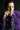New Project! CreativeFlow.ai 🎥 Export GIFs and Videos from Figma

# Michael Yagudaev# Comparing Object in Javascript

As you start writing unit-test you will undoubtedly discover the challenges of comparing object in Javascript. In this short article we will look at how to do object comparison in Javascript to make our unit tests easier to work with. We will take a look at one popular unit-testing framework, Mocha.js, but the lessons here can be applied to other frameworks like QUnit and JSTestDriver as well.

Lets begin by taking a look by considering a task we would like to create tests for.

``````function normalizeYear(yearStr) {
var yearFragments = yearStr.trim().split("-"),
yearRange = _.map(yearFragments, function (p, i) {
var year = parseInt(p, 10);

if(p.trim().match(/\+\$/) && i >= 1) {
year = (new Date()).getFullYear();
}

return isNaN(year) ? null : year <= 99 ?
year > 30 ? 1900 + year : 2000 + year
: year;
});

return yearFragments.length === 2 && yearRange && yearRange ?
_.range(yearRange, yearRange + 1) :
yearStr.trim().match(/\+\$/) ? _.range(yearRange, (new Date()).getFullYear() + 1) : yearRange;
}``````

The function above takes a string representing a range of years and returns an array of the individual years in that range. Pretty simple. Now lets write our first test for it:

``````describe('Utilities', function(){
assert.equal(utils.normalizeYear('2010-2012')), [2010, 2011, 2012]), 'converting a range'); // not going to work since references are compared, not values
});``````

The test above will always fail since assert.equals uses a simple comparision operator (==) to compare the two objects given to it. In our case it is two arrays, each with a different memory reference (memory address). Since the compare in javascript compare the memory addresses it will always return false. Try it by typing `[] == []` in your console (Node.js console or browser console).

Lets try another function assert in Node.js offers us called `assert.deepEqual()`.

``````describe('Utilities', function(){
assert.deepEqual(utils.normalizeYear('2010-2012')), [2010, 2011, 2012]), 'converting a range'); // this works well but does not give good feedback when things break.
});``````

This actually works well. The problem is that when things break you don't get very good feedback on what went wrong. It will simply say: "AssertionError: converting a range", which does not give us much to go on.

Mocha.js like other unit-testing frameworks have a good string compare tool that shows the differences between two strings that are compared using assert.equal. We can use that to our advantage to generate a string from the object and compare them as strings. This will allow us to see the differences between two objects easily. This comes in very handy when you have large objects were you can immediately see why your unit test has failed.

We can do it using the following code:

``assert.equal(JSON.stringify(utils.normalizeYear('2010-2012')), JSON.stringify([2010, 2011, 2012]), 'converting a range');``

This works well, if you can be guranteed the order of keys in your hashes. In our case it is an array with keys 0, 1, 2. The order is guranteed since it is the nature of arrays. But the following test will fail since the order is different:

``    assert.equal(JSON.stringify({ firstname: 'Thomas', lastname: 'Anderson' }), JSON.stringify({ lastname: 'Andreson', firstname: 'Thomas'}));``

This fails because the resulting strings will have the properties in different order. To fix this we will use the following code:

``````/**
* A better way to compare two objects in Javascript
**/
function getKeys(obj) {
var keys;
if(obj.keys) {
keys = obj.keys();
} else {
keys = [];

for(var k in obj) {
if(Object.prototype.hasOwnProperty.call(obj, k)) {
keys.push(k);
}
}
}

return keys;
}

/**
* Create a new object so the keys appear in the provided order.
* @param {Object} obj The object to be the base for the new object
* @param {Array} keys The order in which properties of the new object should appear
**/
function reconstructObject(obj, keys) {
var result = {};
for (var i = 0, l = keys.length; i < l; i++) {
if (Object.prototype.hasOwnProperty.call(obj, keys[i])) {
result[keys[i]] = obj[keys[i]];
}
}

return result;
}

function assertObjectEqual(a, b, msg) {
msg = msg || '';
if( Object.prototype.toString.call( a ) === '[object Array]' && Object.prototype.toString.call( b ) === '[object Array]') {
// special case: array of objects
if (a.filter(function(e) { return Object.prototype.toString.call( e ) === '[object Object]' }).length > 0 ||
b.filter(function(e) { return Object.prototype.toString.call( e ) === '[object Object]' }).length > 0 ){

if (a.length !== b.length) {
assert.equal(JSON.stringify(a), JSON.stringify(b), msg);
} else {
for(var i = 0, l = a.length; i < l; i++) {
assertObjectEqual(a[i], b[i], msg + '[elements at index ' + i + ' should be equal]');
}
}
// simple array of primitives
} else {
assert.equal(JSON.stringify(a), JSON.stringify(b), msg);
}
} else {
var orderedA = reconstructObject(a, getKeys(a).sort()),
orderedB = reconstructObject(b, getKeys(b).sort());

// compare as strings for diff tolls to show us the difference
assert.equal(JSON.stringify(orderedA), JSON.stringify(orderedB), msg);
}
}``````

(Find it on SnippetSky: http://www.snippetsky.com/snippets/5037d0879fcb0c0200000046)

To make sure the order of the keys does not matter, the above code sorts the keys first and then does a string compare based on the sorted keys. This means that doing the following will pass:

``assertObjectEqual({ firstname: 'Thomas', lastname: 'Anderson' }, { lastname: 'Anderson', firstname: 'Thomas'});``

## Recap

Use the code above when you are comparing objects and arrays. If the class of the object is important to you (for dates for example) compare the classes too using the `instanceof` operator in a separate test case.

Happy testing :).

Update 1: Improved assertObjectEqual to support an array of objects as well as just an array of primitives. Update 2: Fixed problem with false positive reports due to a bug in the keys function.